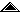Universidad de Costa Rica Centro de Investigación en Matemática Pura y Aplicada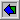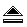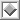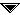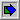# Park-Miller-Carta Random Number Generator in Fortran

## AbstractThe Park and Miller "minimum standard" pseudo random number generator is widely used because of its simple implementation. The improvement suggested by Carta yields a faster linear congruential generator that uses no division. The Fortran implementation provided here complements the already available C and C++ versions. El "estándar mínimo" para generar números seudo aleatorios desarrollado por de Park y Miller es ampliamente utilizado debido a su sencilla implementación. La mejora sugerida por Carta produce un generador congruencial lineal más rápido que no utiliza división. La implementación Fortran aquí proporcionada complementa las ya disponibles en C y C++.

## MotivationWhen Park & Miller developed their "minimal standard" for a linear congruential [LCG] pseudo random number generator [PRNG] they succeeded in providing a "good enough" LCG for "the rest of us" [PM-1998]. In their paper, they provided a Pascal implementation that can be easily translated into C or C++, as the basic formula for the LCG is simple: `(16807 mod 2^32 -1)`. Later on, Carta suggested and improvement of the algorithm that uses no division [Carta-1990]. A good explanation of Carta's improvement can be found in [Whittle-2013], where a Fortran implementation was promised. That promise gets fulfilled in here.

 ```#include int32_t next_16807_64b( int32_t seed ) { enum { two_31_1 = 2147483647 }; int64_t next = seed; next = ( ( next * (int64_t)(16807) ) % two_31_1 ); return (int32_t)(next); } ```
Figure 1

Figure 1 is a straightforward implementation of the "minimal standard". It has two defects: uses 64 bit arithmetic and division. This LCG will generate every number in range "`[1,...,2^31-2]`" exactly once: this is a full period generator.

## Schrage's improvementIn [Schrage-1987] a simple procedure is provided to calculate the result of a multiplication using modulo arithmetic. This trick is based on the Quotient/Remainder theorem that states that for any integer "`m`" and "`a>0`" there exist unique integers "`q`" (quotient) and "`r`" (remainder) such that "`m = a*q+r`" and "`0≤r<m`".

This theorem was applied by Schrage to avoid overflow when calculating the modulo result of an integer multiplication [Bathsheba-2014] . Suppose you want to calculate the multiplication "`a*z mod m`". First, decompose the modulus "`m>0`" using the multiplier "`a`": "`m = a*q+r`". This means that "`q=[m/a]`" and "`r = m mod a`", where [] is the integer part. It does not always happen, but suppose that "`r<q`"and "`0<z<m-1`" then both "`a*(z mod q)`" and "`r*[z/q]`" will be in range "`[0,...,m-1]`". Furthermore, "`a*z mod m = a*(z mod q) - r*[z/q]`". If this difference is negative just add "`m`".

 ```function Random32 : real; (* Integer Version 2 *) const a = 16807; m = 2147483647; q = 127773; (* m div a *) r = 2836; (* m mod a *) var lo, hi, test : integer; begin hi := seed div q; lo := seed mod q; test := a * lo - r * hi; if test > 0 then seed := test else seed := test + m; Random32 := seed / m end; ```
Figure 2

The implementation in Figure 2 is the original implementation provided by Park & Miller in their paper, where they use Schrage's trick to calculate "`a*z mod m`". No overflow will occur and 32 bit arithmetic is used, but division is still used in the algorithm.

## Carta's implementationTo avoid using divisions, Carta's implementation of the "minimal standard" uses only multiplications [Carta-1990]. His explanation is a little difficult to follow, but in a latter paper Whittle provides a simpler explanation [Whittle-2013].

 ```/* Park-Miller "minimal standard" 31 bit * pseudo-random number generator, implemented * with Davis G. Carta's optimisation: with * 32 bit math and without division. */ long unsigned int rand31_next() { long unsigned int lo, hi; lo = 16807 * (seed & 0xFFFF); hi = 16807 * (seed >> 16); lo += (hi & 0x7FFF) << 16; lo += hi >> 15; if (lo > 0x7FFFFFFF) lo -= 0x7FFFFFFF; return ( seed = (long) lo ); } ```
Figure 3

The implementation in Figure 3 is taken from Whittle's contribution. It no longer uses division and relies on 32 bit unsigned arithmetic. To test that it does produce the correct result, its output can be compared with the original algorithm in Figure 2; on current machines, this takes little time (less than a few minutes). 2's complement arithmetic is assumed in this implementation (a ternary computer might not produce the correct result).

## Fortran issuesWhen you have years of C++ programming, it becomes hard to produce Fortran implementations. However, it is easy enough to translate Carta's C implementation into Fortran, but small difficulties arise. When testing whether variable "`lo`" is negative, the C language test uses a comparison to "`0x7FFFFFFF`" ( 2^31-1 ). As Fortran integers are signed the unsigned integer test in C cannot be directly transliterated to Fortran: another test that achieves the same result must be used.

 ``` subroutine pmc_next( SEED ) integer(4) SEED integer(4) lo, hi lo = 16807 * IAND( SEED, 65535_4 ) ! Z'FFFF' = 65535 hi = 16807 * ISHFT(SEED,-16) lo = lo + ISHFT(IAND(hi,32767_4),16) ! Z'7FFF' = 32767 lo = lo + ISHFT(hi,-15) if ( lo .LT. 0 ) then ! 2147483647==Z'7FFFFFFF' lo = lo-2147483647_4 endif SEED = lo return end subroutine ```
Figure 4

When 2's complement is used in computers, the first bit of an integer number would be `'1'` when the stored value is negative. Hence, instead of asking whether the "`0x7FFFFFFF`" barrier was crossed, the signed equivalent is to test for a negative value. This is apparent in the Fortran implementation of Figure 4. The underscored suffix digit in Fortran denotes the constant byte size: "`2147483647_4`" is a 4 byte integer value (32 bits) and "`2147483647_8`" is an 8 byte integer value (64 bits).

 ``` Program test_rand31pmc c ---------------------- integer(4) SEED integer(4) next_fortran, next_cpp write (*,'(A)') 'BEGIN' do SEED = 1 , 2147483647_4-1 next_fortran = SEED; call pmc_next( next_fortran ); next_cpp = SEED; call pm_next( next_cpp ); if ( next_fortran .NE. next_cpp ) then write (*,'(I10,A,I0,A,I0)') c SEED,' -> ',next_cpp,'.NE.',next_fortran  call sleep(1*0) endif enddo write (*,'(A)') 'END' if (.true.) then write (*,'(I0,A,I10,A)') c sizeof(2147483647_4),'->int(4)', c sizeof(2147483647_8),'->int(8)' endif end ! test_rand31pmc ```
Figure 5

Testing the Fortran implementation for correctness is done with a Fortran main program linked with the C language version of the Park & Miller algorithm. A simple loop through every possible value test that the Fortran implementation always produces the correct value, as shown in Figure 5.

Some other issues had to be tackled to mix Fortran and C, but the problems rise when using an Integrated Development Environment [IDE]. In this case Code::Blocks version 16.01 was used, but it is a little stiff for mixing modules from different languages. In the Windows platform, the Code::Blocks Fortran project file (with extension `.cbp`) required to use a direct path to invoke the C compiler:

```<Option
compiler="gfortran"
use="1"
buildCommand='&quot;%ProgramFiles%\CodeBlocks\MinGW\bin\gcc.exe&quot; -g -c \$file -o \$object'
/>
```

Fewer problems arise when invoking the Fortran and C compilers from the command line, as Unix/Linux people usually do. A good guide with more information on mixed language programming is [Ippolito-2016].

## ConclusionsThe "minimal standard" LCG has many qualities. Implementation that use Carta's speed up help choosing it for many applications where "cryptographically secure" PRNG are not required.

## AcknowledgmentsAlejandro Di Mare made valuable suggestions that helped improve earlier versions of this work. The Centro de Investigación en Matemática Pura y Aplicada [CIMPA] at the Universidad de Costa Rica provided resources for this research. The spelling and grammar were checked using the `http://spellcheckplus.com/` tool.

## References[Bathsheba-2014] Bathsheba: Modular multiplication of large numbers in c++, 2014.       `http://stackoverflow.com/questions/20971888/#20972369` [Carta-1990] Carta, David G.: Two Fast Implementations of the "Minimal Standard" Random Number Generator, 1990.       `http://www.firstpr.com.au/dsp/rand31/p87-carta.pdf` [Ippolito-2016] Ippolito, Greg: Using C/C++ and Fortran together, 2001-2016.       `http://www.yolinux.com/TUTORIALS/LinuxTutorialMixingFortranAndC.html` [PM-1998] Park, Stephen K. & Miller, Keith W.: Random Number Geuerators: Goodones Are Hard To Find, Communications of the ACM, Oct 1988, Vol 31 Number 10 1192-1201.       `http://www.firstpr.com.au/dsp/rand31/p1192-park.pdf` [Schrage-1987] Bratley, Paul & Fox, Bennet L. & Schrage, Linus E.: A Guide to Simulation 2ed, ISBN-10: 0387964673, ISBN-13: 978-0387964676, Springer 1987. [Whittle-2013] Whittle, Robin: Park-Miller-Carta Pseudo-Random Number Generator, 2005-2013.       `http://www.firstpr.com.au/dsp/rand31` `           `

## IndexMotivation  Schrage's improvement  Carta's implementation  Fortran issues  Conclusions  Acknowledgements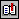ReferencesIndex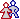About the author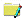About this documentBeginIndexEnd

## About the author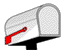Adolfo Di Mare <adolfo@di-mare.com>
## About this documentReferencia: Di Mare, Adolfo: Park-Miller-Carta Random Number Generator in Fortran: Technical Report 2016-02-ADH, Centro de Investigación en Matemática Pura y Aplicada [CIMPA], Universidad de Costa Rica, 2016. Internet: ``` http://www.di-mare.com/adolfo/p/rand31pmc.htm ```       Google™ Translate ``` http://www.di-mare.com/adolfo/p/src/rand31pmc.zip ``` Author: Adolfo Di Mare ``` ``` Contact: Apdo 4249-1000, San José Costa Rica Tel: (506) 2511-6606       Fax: (506) 2511-4918 Revision: CIMPA, September 2016 Visitantors: